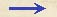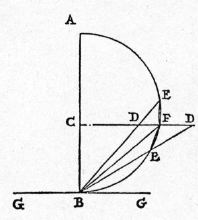Discorsi Propositions 2/35-pr-14-lemma-1 Discorsi Proposition2/35-pr-14-lemma-1{260} LEMMA. {260} LEMMASit DC ad diametrum BA perpendicularis, et a termino B educatur BED utcunque, et connectatur FB: dico; FB inter DG, BE esse mediam. Let DC be drawn perpendicular to the diameter BA; from the extremity B draw the line BED at random; draw the line FB. Then, I say, FB is a mean proportional between DB and BE. Connectatur EF, et per B ducatur tangens BG, quae erit ipsi CD parallela; quare angulus DBG angulo FDB erit aequalis: at eidem GBD aequatur quoque angulus EFB in portione alterna: ergo similia sunt triangula FBD, FEB, et ut BD ad BF, ita FB {10} ad BE. Join the points E and F. Through B, draw the tangent BG which will be parallel to CD. Now, since the angle DBG is equal to the angle FDB, and since the alternate angle of GBD is equal to EFB, it follows that the triangles FDB and FEB are similar and hence BD:BF = FB:BE.Discorsi Propositions 2/35-pr-14-lemma-1 Discorsi Proposition2/35-pr-14-lemma-1